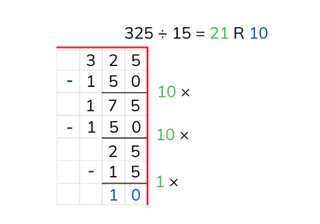Partial quotients division with a number to 1,000 with remainder

# Partial quotients division with a number to 1,000 with remainder

Students learn partial quotients division with a number to 1,000 with remainder.

No account needed.8,000 schools use Gynzy92,000 teachers use Gynzy1,600,000 students use Gynzy

## General

The students learn partial quotients division with a number to 1,000 with remainder.

## Standards

CCSS.Math.Content.5.NBT.B.5

## Learning objective

Students will be able to use partial quotients to divide a number to 1,000 with remainder.

## Introduction

Throw a die. The number of dots gives which problem the students must solve. Repeat this a number of times.

## Instruction

Explain that with partial quotients division you break up the problem into pieces and solve it in this way. You write down the problem and then draw a line underneath. Next you can use a helping row from the table of the number that you are dividing by. In this row you don't have to write down every problem from the table. You can double and halve problems, or put a 0 at the end. After this you solve the problem step by step to see how many times 16 fits in 421. After every step you write down how much is left over. Sometimes you still have a number left over that can no longer be divided by 16. This number is your remainder. Next you add up all of the numbers that tell how many times 16 fits in 421 (20, 5, and 1) and give how much you have left over (5). Explain that you will sometimes be asked for remainder, but there is no remainder in the problem. In this case you write a 0 as the remainder. Have the students solve a few problems using partial quotients division. Have them write the problem down on graphing paper, and they may also write down a helping row. Ask various students what steps they took to solve the problems. Encourage the students to take as large steps as they are able.

Check whether the students can use partial quotients to divide by asking the following questions:
- Why is it useful to be able to divide using partial quotients?
- What aid can you use when dividing using partial quotients?
- Solve this problem using partial quotients division: 359 ÷ 16

## Quiz

The students test their understanding of partial quotients division with a number to 1,000 with remainder through ten exercises. In each exercises they must solve the division problem using partial quotients, and give the remainder.

## Closing

You discuss once again with the students how it is useful to be able to divide using partial quotients with a number to 1,000 with remainder, because that is how you can solve problems in an easier way. Check if the students know that with partial quotients division you solve parts of the problem in steps and that they can use a helping row to help with their calculations. Have the students name a number to 1,000 for the first number. Then you spin the wheel for the second number and the students must solve the resulting division problem using partial quotients division.

## Teaching tips

When students have difficulty with partial quotients division, you can have them make helping rows of the table of the number that they are dividing by. First have them solve a problem step by step. Look carefully at the steps they make, and try to encourage them to make larger steps (not the step 10 × 3 = 30 ten times, but in one step 100 × 3 = 300). First practice with a one-digit number. Then have the students practice partial quotients division by a number that consists of two digits.

## Instruction materials

Graphing paper

### The online teaching platform for interactive whiteboards and displays in schools

• Save time building lessons

• Manage the classroom more efficiently

• Increase student engagement